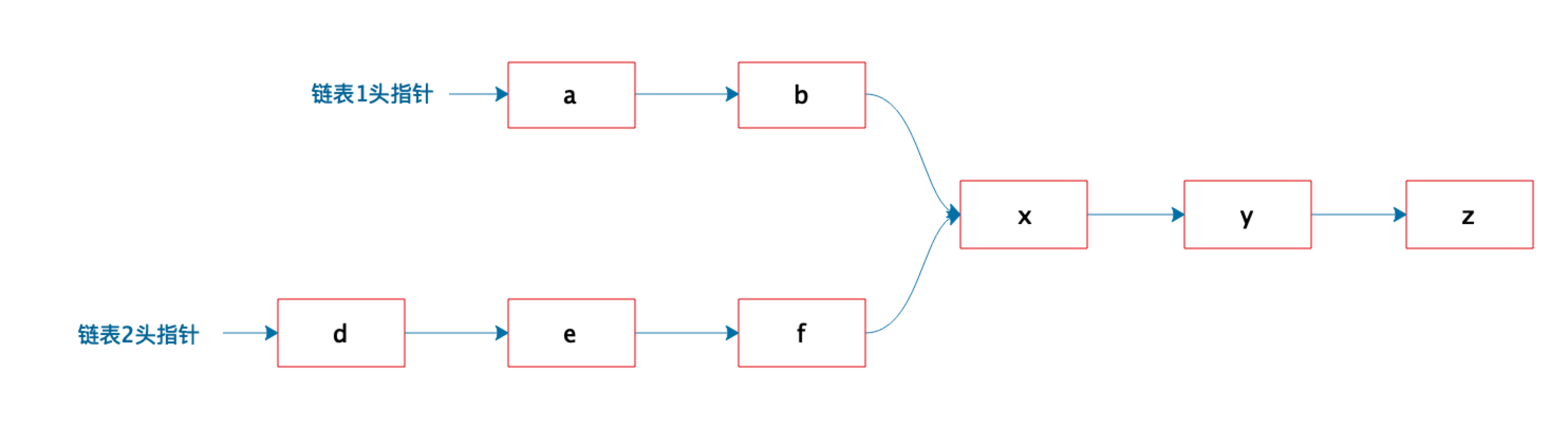# 架构师训练营第 8 周作业 算法与数据结构TH

﻿﻿

#### 方法一

def find_intersection(chain_a, chain_b):    node_a = chain_a.start    node_b = chain_b.start    if node_a == node_b:        print 'intersect at', node_a        return    while node_a.next:        while node_b.next:            if node_a == node_b:                print 'intersect at', node_a                return    print 'no intersection'

﻿

#### 方法二

def find_intersection(chain_a, chain_b):    node_a = chain_a.start    node_b = chain_b.start     if node_a == node_b:        print 'intersect at', node_a        return		    stack_a = Stack()    stack_b = Stack()    stack_a.put(node_a)    stack_b.put(node_b)    while node_a.next:        node_a = node_a.next        stack_a.put(node_a)    while node_b.next:        node_b = node_b.next        stack_b.put(node_b)    last = None    while True:        if stack_a.is_empty() or stack_b.is_empty():            break        node_a = stack_a.pop()        node_b = stack_b.pop()        if node_a == node_b:            last = node_a            continue        if node_a != node_b:            break    if last:        print 'intersect at', last    else:        print 'no intersection'

﻿

#### 方法三

def find_intersection(chain_a, chain_b):    node_a = chain_a.start    node_b = chain_b.start    if node_a == node_b:        print 'intersect at', node_a        return    if len(chain_a) >= len(chain_b):        step = len(chain_a) - len(chain_b)        for i in range(step):            node_a = node_a.next    else:        step = len(chain_b) - len(chain_a)        for i in range(step):            node_b = node.next    while node_a.next:        if node_a.next == node_b.next:            print 'intersect at', node_a.next            return        node_a = node_a.next        node_b = node_b.next    print 'no intersection'

﻿

#### 方法四

def find_intersection(chain_a, chain_b):    node_a = chain_a.start    node_b = chain_b.start    if node_a == node_b:        print 'intersect at', node_a        return    hash_table = {}    while node_a.next:        hash_table[hash(node_a.addr)] = node_a        node_a = node_a.next    while node_b.next:        if hash_table[hash(node_b.addr)]:            print 'intersect at', node_b            return        node_b = node_b.next    print 'no intersection'

﻿

﻿

﻿

﻿

﻿## 评论